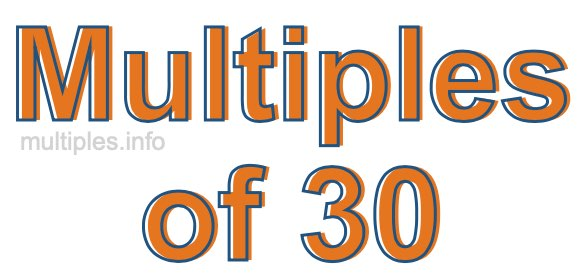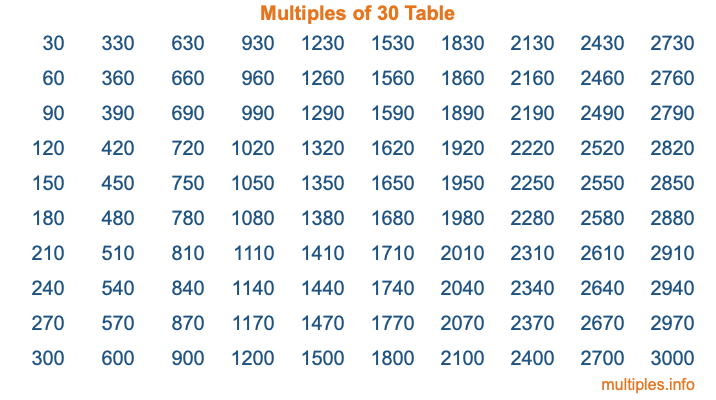Multiples of 30Welcome to the Multiples of 30 page. Here we will first teach you everything you will ever need to know about the multiples of 30, and then give you a study guide summary of everything we taught you to make sure you remember it all. Use this page to look up facts and learn information about the multiples of 30. This page will make you a multiples of thirty expert!

Definition of Multiples of 30
Multiples of 30 are all the numbers that when divided by 30 equal an integer. Each of the multiples of 30 are called a multiple. A multiple of 30 is created by multiplying 30 by an integer.

Therefore, to create a list of multiples of 30, you start with 1 multiplied by 30, then 2 multiplied by 30, then 3 multiplied by 30, and so on for as long as you want. Thus, the list of the first five multiples of 30 is 30, 60, 90, 120, and 150. To see a larger list of multiples of 30, see the printable image of Multiples of 30 further down on this page. We also have a category where you can choose any nth multiple of 30.

Multiples of 30 Checker
The Multiples of 30 Checker below checks to see if any number of your choice is a multiple of 30. In other words, it checks to see if there is any number (integer) that when multiplied by 30 will equal your number. To do that, we divide your number by 30. If the the quotient is an integer, then your number is a multiple of 30.

Is  a multiple of 30?

Least Common Multiple of 30 and ...
A Least Common Multiple (LCM) is the lowest multiple that two or more numbers have in common. This is also called the smallest common multiple or lowest common multiple and is useful to know when you are adding our subtracting fractions. Enter one or more numbers below (30 is already entered) to find the LCM.

Check out our LCM Calculator if you need more details about the Least Common Multiple or if you need the LCM for different numbers for adding and subtraction fractions.

nth Multiple of 30
As we stated above, 30 is the first multiple of 30, 60 is the second multiple of 30, 90 is the third multiple of 30, and so on. Enter a number below to find the nth multiple of 30.

th multiple of 30

Multiples of 30 vs Factors of 30
30 is a multiple of 30 and a factor of 30, but that is where the similarities end. All postive multiples of 30 are 30 or greater than 30. All positive factors of 30 are 30 or less than 30.

Below is the beginning list of multiples of 30 and the factors of 30 so you can compare:

Multiples of 30: 30, 60, 90, 120, 150, etc.

Factors of 30: 1, 2, 3, 5, 6, 10, 15, 30

As you can see, the multiples of 30 are all the numbers that you can divide by 30 to get a whole number. The factors of 30, on the other hand, are all the whole numbers that you can multiply by another whole number to get 30.

It's also interesting to note that if a number (x) is a factor of 30, then 30 will also be a multiple of that number (x).

Multiples of 30 vs Divisors of 30
The divisors of 30 are all the integers that 30 can be divided by evenly. Below is a list of the divisors of 30.

Divisors of 30: 1, 2, 3, 5, 6, 10, 15, 30

The interesting thing to note here is that if you take any multiple of 30 and divide it by a divisor of 30, you will see that the quotient is an integer.

Multiples of 30 Table
Below is an image of the first 100 multiples of 30 in a table. The table is in chronological order, column by column. The first column has the first ten multiples of 30, the second column has the next ten multiples of 30, and so on.The Multiples of 30 Table is also referred to as the 30 Times Table or Times Table of 30. You are welcome to print out our table for your studies.

Negative Multiples of 30
Although not often discussed or needed in math, it is worth mentioning that you can make a list of negative multiples of 30 by multiplying 30 by -1, then by -2, then by -3, and so on, to get the following list of negative multiples of 30:

-30, -60, -90, -120, -150, etc.

Multiples of 30 Summary
Below is a summary of important Multiples of 30 facts that we have discussed on this page. To retain the knowledge on this page, we recommend that you read through the summary and explain to yourself or a study partner why they hold true.

There are an infinite number of multiples of 30.

A multiple of 30 divided by 30 will equal a whole number.

30 divided by a factor of 30 equals a divisor of 30.

The nth multiple of 30 is n times 30.

The largest factor of 30 is equal to the first positive multiple of 30.

30 is a multiple of every factor of 30.

30 is a multiple of 30.

A multiple of 30 divided by a divisor of 30 equals an integer.

30 divided by a divisor of 30 equals a factor of 30.

Any integer times 30 will equal a multiple of 30.

Multiples of a Number
Here you can get the multiples of another number, all with the same attention to detail as we did for multiples of 30 on this page.

Multiples of
Multiples of 31
Did you find our page about multiples of thirty educational? Do you want more knowledge? Check out the multiples of the next number on our list!